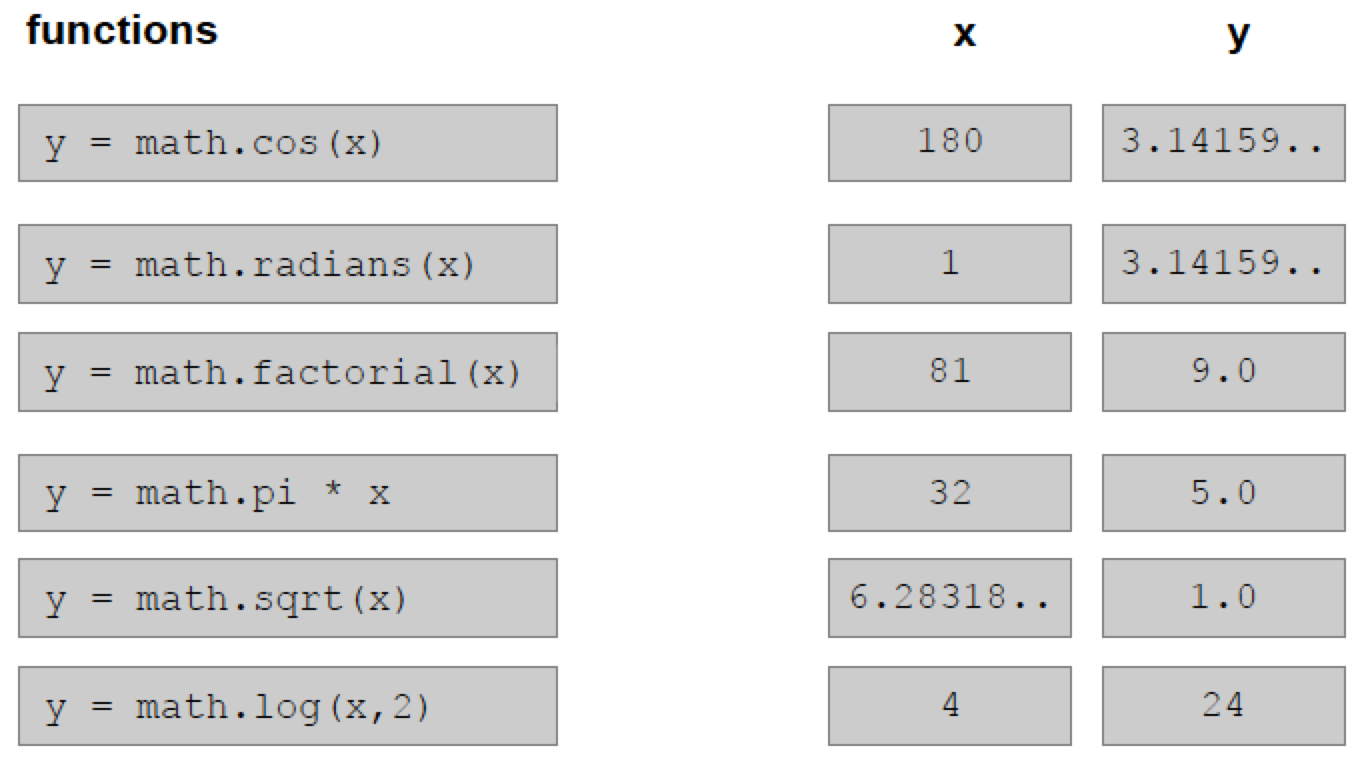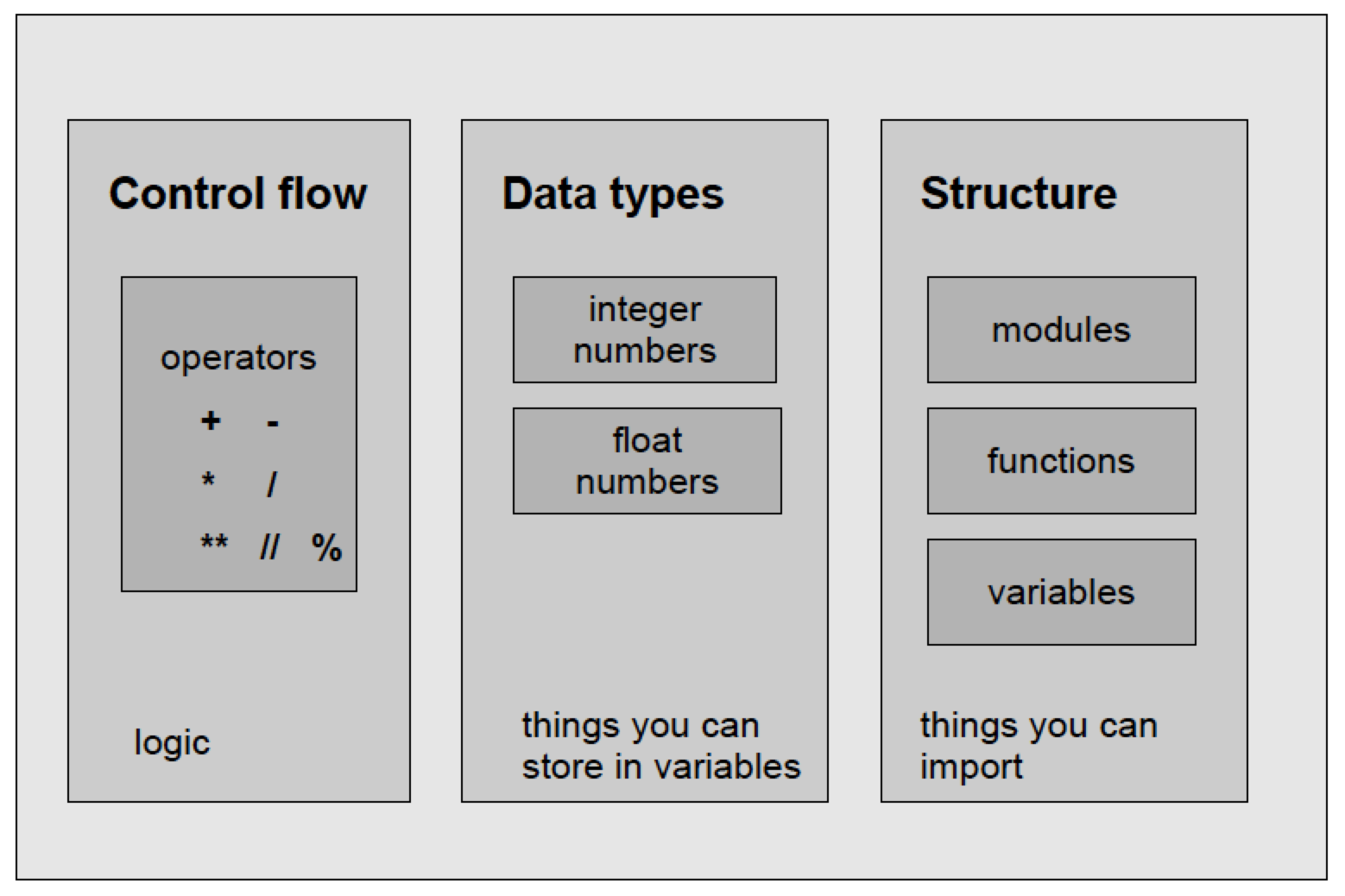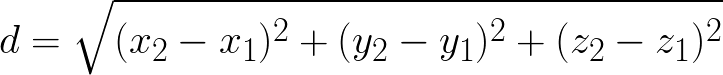In this module you can learn:

• How to use Python as a pocket calculator
• How to store data in variables
• How to import functions from Python modules

### Traces in the desert sand…Python can be used as a calculator:

``````>>> 1+2
>>> 3
``````

Fill in dashed lines with appropriate values using the python interactive shell

``````>>> 1 _ _ 2
>>> 3
>>> 12 - _ _
4
>>> _ _ * 5
20
>>> _ _ ** 2
81
``````

Python can be used to store variables:

``````>>> camels = 9
>>> camels
_ _
>>> silk = camels *  _ _
>> silk
36
>>> math.sqrt(silk)
_ _
>>> math.pow(camels, _ _ )
81.0
``````

### The interactive Python shell

You can access the shell by typing `python` in a command-line terminal

``````\$ python
Python 2.7.9 (default, Apr  2 2015, 15:33:21)
[GCC 4.9.2] on linux2
>>>
``````

You can leave the shell with `Ctrl-D`

Challenge #1

Open a Python interactive session (the Python shell):

• Calculate the sum and difference of two numbers
• Divide two numbers. Try 5/3.
• Then try 5.0/3
• Calculate 3x5
• Calculate a power of 2

### Variables

Variables are containers for data.

Variable names may be composed of letters, underscores and, after the first position, also digits.

``````>>> camels = 9
>>> camels
9
>>> silk = camels * 4
>> silk
36
``````

### The math module

Sometimes you need more complex mathematical constants and functions

• square root
• factorial
• sine or cosine
• pi
• log

Python groups them together in a text file. You can access them by importing the file.

``````import math
``````

Find the matching pairs of functions and x/y values.### Components of Python### The Dogma of Programming

• First, make it work.
• Second, make it nice.
• Third, and only if it is really necessary, make it fast.

Challenge #2

• The diameter of a cell is 10 μm.
• What volume does that cell have given it is a perfect sphere?
• Use Python to do the calculation.
• Use variables for the parameters.
• Print the volume to the screen

See the Solution to challenge #2

Challenge #3

Calculate the distance between two points in the 3D space

Given two points in the Cartesian space:

P1 = (43.64, 30.72, 88.95) P2 = (45.83, 31.11, 92.04)

• Use Python to calculate their distance
• Use variables for the parameters
• Print the distance to the screen

The formula for calculating the distance is:See the Solution to challenge #3

Challenge #4

Find cysteine bonds in the Insulin structure

• Data in 2OMH.pdb
• Lines containing ‘SG’
• x, y, z coordinates
• Use what you learnt from Task 2

``````HEADER    HORMONE                                 22-JAN-07   2OMH
TITLE     STRUCTURE OF HUMAN INSULIN COCRYSTALLIZED WITH ARG-12 PEPTIDE IN
TITLE    2 PRESENCE OF UREA
COMPND    MOL_ID: 1;
COMPND   2 MOLECULE: INSULIN A CHAIN;
COMPND   3 CHAIN: A
COMPND   4 MOL_ID: 2;
COMPND   5 MOLECULE: INSULIN B CHAIN;
COMPND   6 CHAIN: B
ATOM      1  N   GLY A   1     -11.626  14.280   1.013  1.00 19.13           N
ATOM      2  CA  GLY A   1     -12.164  13.430   2.116  1.00 18.24           C
ATOM      3  C   GLY A   1     -11.587  13.815   3.470  1.00 17.90           C
ATOM      4  O   GLY A   1     -10.907  14.833   3.590  1.00 18.08           O
ATOM      5  N   ILE A   2     -11.836  12.995   4.484  1.00 17.57           N
ATOM      6  CA  ILE A   2     -11.397  13.317   5.832  1.00 17.78           C
ATOM      7  C   ILE A   2      -9.875  13.489   5.951  1.00 17.93           C
ATOM      8  O   ILE A   2      -9.408  14.371   6.670  1.00 17.80           O
ATOM      9  CB  ILE A   2     -11.922  12.289   6.869  1.00 17.86           C
ATOM     10  CG1 ILE A   2     -11.767  12.855   8.281  1.00 17.66           C
ATOM     11  CG2 ILE A   2     -11.242  10.918   6.680  1.00 18.86           C
ATOM     12  CD1 ILE A   2     -12.434  12.031   9.365  1.00 19.10           C
ATOM     13  N   VAL A   3      -9.110  12.680   5.224  1.00 18.04           N
ATOM     14  CA  VAL A   3      -7.652  12.759   5.315  1.00 19.40           C
ATOM     15  C   VAL A   3      -7.193  14.102   4.747  1.00 19.43           C
ATOM     16  O   VAL A   3      -6.395  14.820   5.357  1.00 19.23           O
``````

Challenge #5

The hydrolysis of one phosphodiester bond from ATP results in a standard Gibbs energy (ΔG0) of -30.5 kJ/mol. According to biochemistry textbooks, the real ΔG value depends on the concentration of the compounds and these concentrations can differ quite a lot among tissues.

The Gibbs energy as a function of the concentrations of the compounds can be written as:

ΔG = ΔG0 + RT * ln ( [ADP] * [Pi] / [ATP])

Knowing that:

R = 0.00831

T = 298

• Use Python to calculate the real DG in the tissues reported in the table.
• Use variables for the parameters.
• Print the results to the screen.

See See the Solution to challenge #5

### Recap

• You can use the Python shell as a pocket calculator.
• Variables are containers for data.
• Modules are containers for data and functions
• You can leave the shell by Ctrl-D.

### Back

Back to main page.